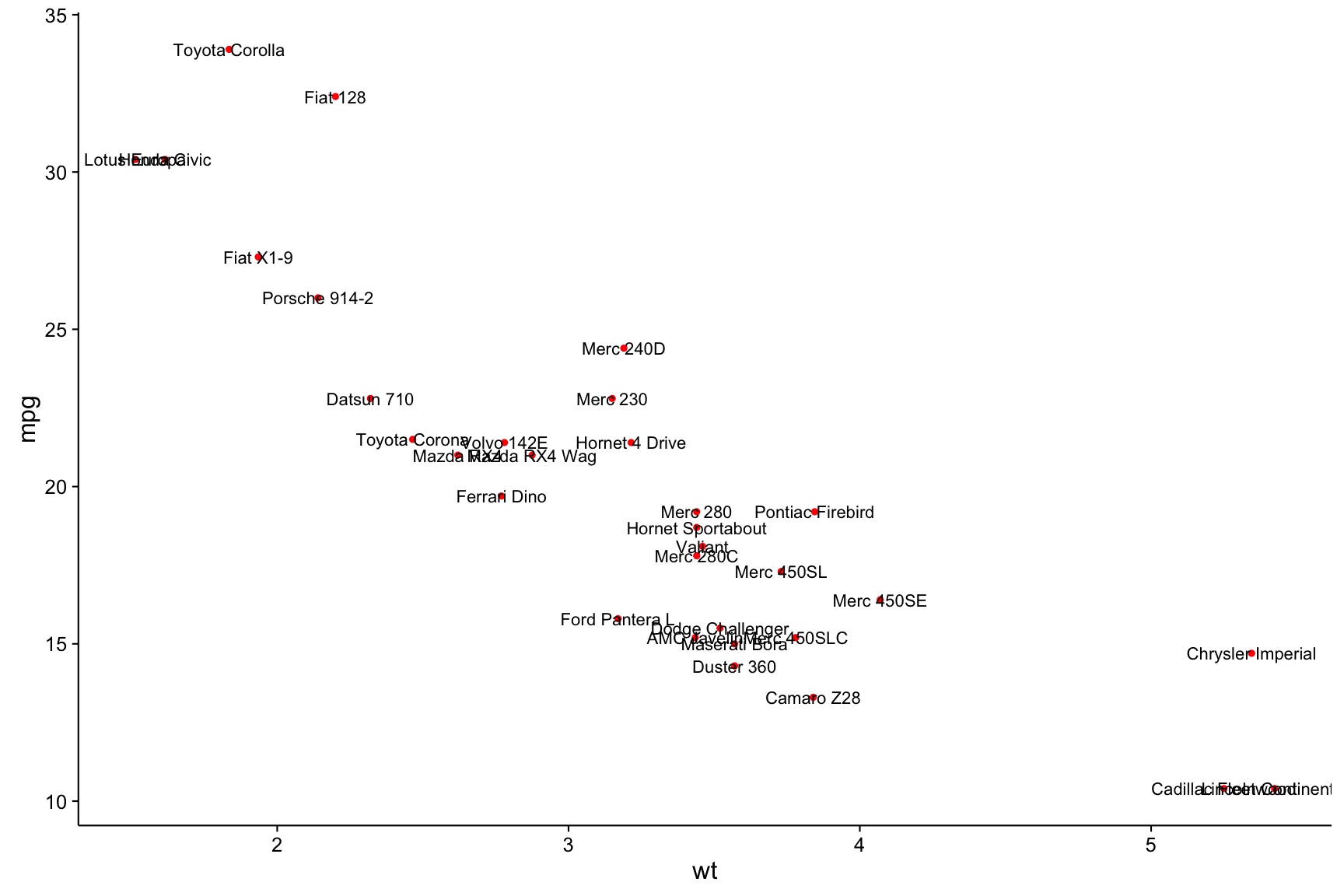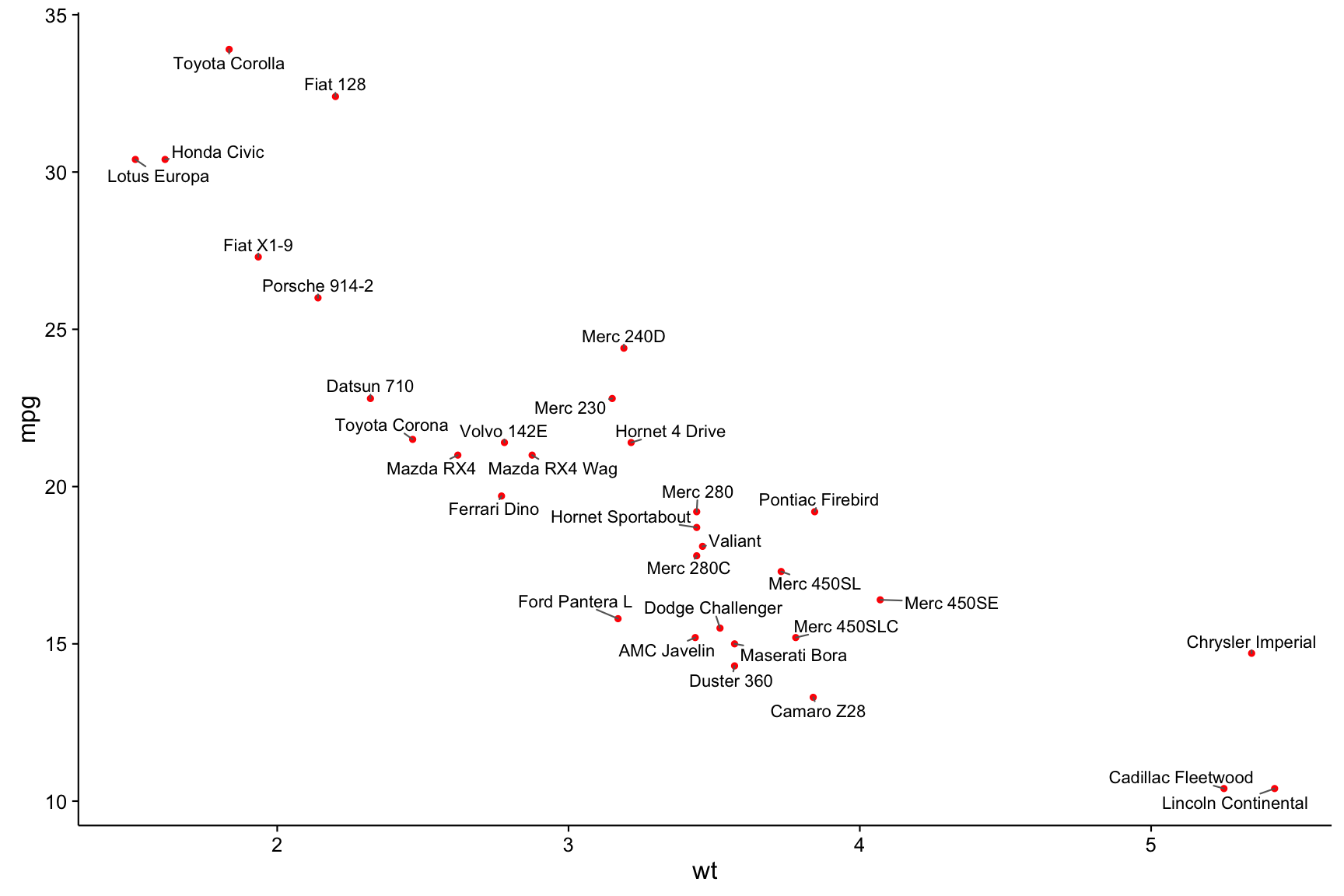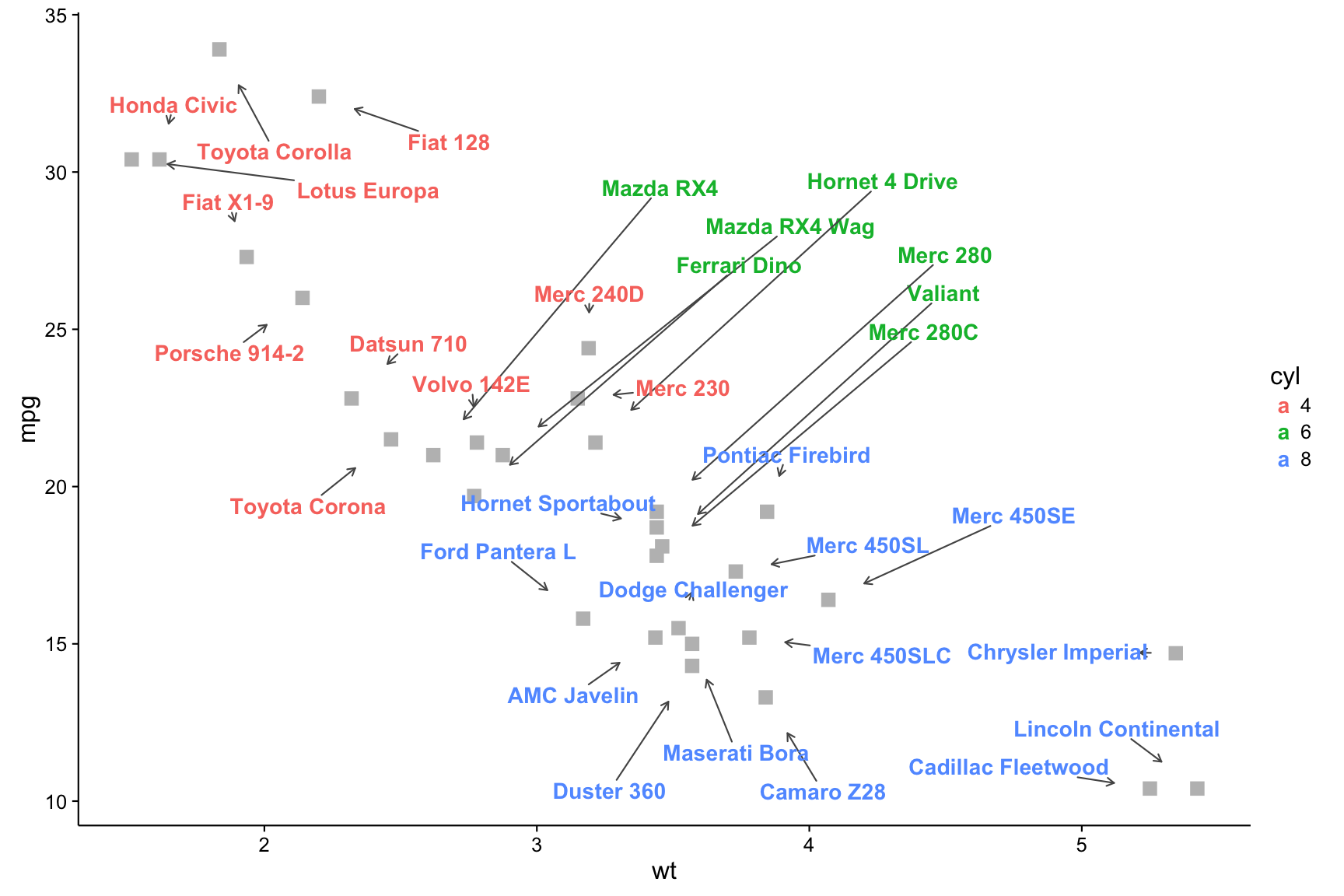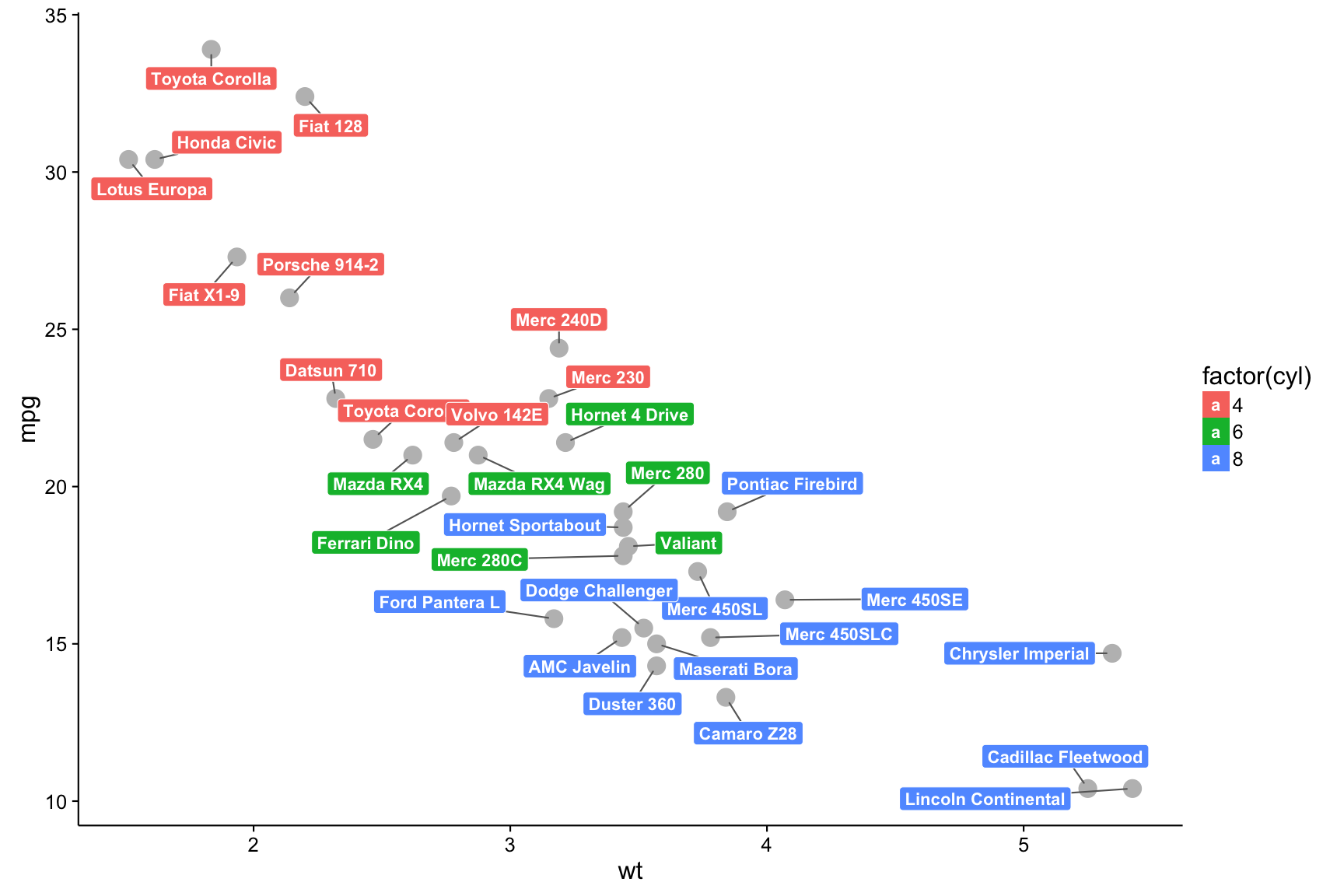# ggrepel

## Motivation

Some text labels overlap each other in plots created with geom_text:

``````library(ggplot2)
ggplot(mtcars) +
geom_point(aes(wt, mpg), color = 'red') +
geom_text(aes(wt, mpg, label = rownames(mtcars))) +
theme_classic(base_size = 16)``````## Algorithm

`ggrepel` implements functions to repel overlapping text labels away from each other and away from the data points that they label. The algorithm works as follows:

• For each box:
• Move the box into the allowed plotting area.
• If the bounding box overlaps other boxes:
• Repel the overlapping boxes from each other.
• If the bounding box overlaps data points:
• Repel the box away from the data points.
• Repeat until all overlaps are resolved, up to a preset limit of iterations.

## Usage Examples

### geom_text_repel

We can repel the text labels away from each other by loading `ggrepel` and using `geom_text_repel` instead:

``````library(ggrepel)
set.seed(42)
ggplot(mtcars) +
geom_point(aes(wt, mpg), color = 'red') +
geom_text_repel(aes(wt, mpg, label = rownames(mtcars))) +
theme_classic(base_size = 16)``````#### Options

All options available for geom_text such as `size` and `fontface` are also available for `geom_text_repel`.

However, the following parameters are not supported:

• `hjust`
• `vjust`
• `position`
• `check_overlap`

`ggrepel` provides additional parameters for `geom_text_repel` and `geom_label_repel`:

• `segment.color` is the line segment color
• `box.padding` is the padding surrounding the text bounding box
• `point.padding` is the padding around the labeled point
• `arrow` is the specification for arrow heads created by `grid::arrow`
• `force` is the force of repulsion between overlapping text labels
• `max.iter` is the maximum number of iterations to attempt to resolve overlaps
• `nudge_x` is how much to shift the starting position of the text label along the x axis
• `nudge_x` is how much to shift the starting position of the text label along the y axis
``````set.seed(42)
ggplot(mtcars) +
geom_point(aes(wt, mpg), color = 'grey', size = 4, shape = 15) +
geom_text_repel(
aes(
wt, mpg,
color = factor(cyl),
label = rownames(mtcars)
),
size = 5,
fontface = 'bold',
segment.color = '#555555',
segment.size = 0.5,
arrow = arrow(length = unit(0.01, 'npc')),
force = 1,
max.iter = 2e3,
nudge_x = ifelse(mtcars\$cyl == 6, 1, 0),
nudge_y = ifelse(mtcars\$cyl == 6, 8, 0)
) +
scale_color_discrete(name = 'cyl') +
theme_classic(base_size = 16)``````### geom_label_repel

`geom_label_repel` is based on geom_label.

``````set.seed(42)
ggplot(mtcars) +
geom_point(aes(wt, mpg), size = 5, color = 'grey') +
geom_label_repel(
aes(wt, mpg, fill = factor(cyl), label = rownames(mtcars)),
fontface = 'bold', color = 'white',
) +
theme_classic(base_size = 16)``````### Line plot

``````set.seed(42)
ggplot(Orange, aes(age, circumference, color = Tree)) +
geom_line() +
coord_cartesian(xlim = c(min(Orange\$age), max(Orange\$age) + 90)) +
geom_text_repel(
data = subset(Orange, age == max(age)),
aes(label = paste("Tree", Tree)),
size = 6,
nudge_x = 45,
segment.color = NA
) +
theme_classic(base_size = 16) +
theme(legend.position = "none") +
labs(x = "Age (days)", y = "Circumference (mm)")``````